单链表的翻转递归和非递归方式（php实现、）

1、最重要的是理解单链表的数据结构，（链表操作一定要画图，画图才能理解的更为深刻。）

1：单向链表：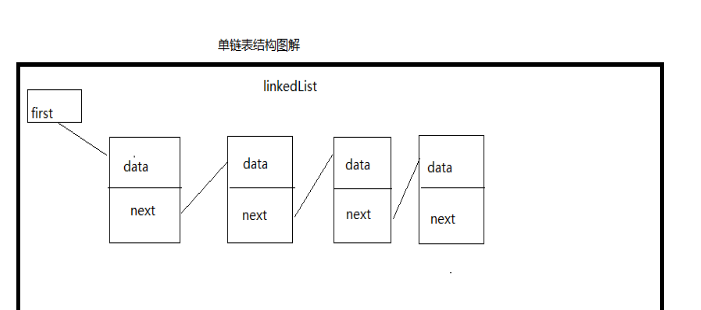2、我们知道迭代是从前往后依次处理，直到循环到链尾；而递归恰恰相反，首先一直迭代到链尾也就是递归基判断的准则，然后再逐层返回处理到开头。总结来说，链表翻转操作的顺序对于迭代来说是从链头往链尾，而对于递归是从链尾往链头。下面我会用详细的图文来剖析其中实现的细节

1）非递归方式（迭代）方式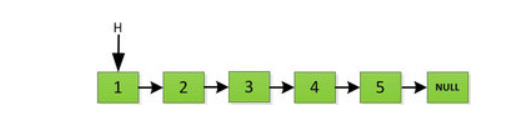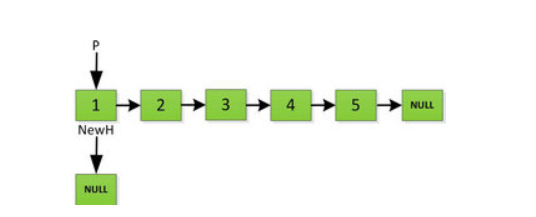然后依次将旧链表上每一项添加在新链表的后面，然后新链表的头指针NewH移向新的链表头，如下图所示。此处需要注意，不可以上来立即将上图中P->next直接指向NewH，这样存放2的地址就会被丢弃，后续链表保存的数据也随之无法访问。而是应该设置一个临时指针tmp，先暂时指向P->next指向的地址空间，保存原链表后续数据。然后再让P->next指向NewH，最后P=tmp就可以取回原链表的数据了，所有循环访问也可以继续展开下去。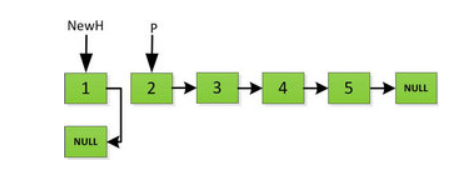指针继续向后移动，直到P指针指向NULL停止迭代。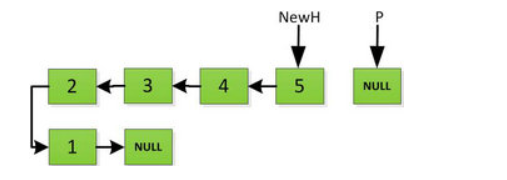2) 递归实现。

我们再来看看递归实现链表翻转的实现，前面非递归方式是从前面数1开始往后依次处理，而递归方式则恰恰相反，它先循环找到最后面指向的数5，然后从5开始处理依次翻转整个链表。
首先指针H迭代到底如下图所示，并且设置一个新的指针作为翻转后的链表的头。由于整个链表翻转之后的头就是最后一个数，所以整个过程NewH指针一直指向存放5的地址空间。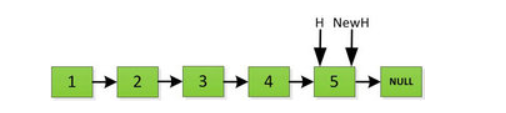然后H指针逐层返回的时候依次做下图的处理，将H指向的地址赋值给H->next->next指针，并且一定要记得让H->next =NULL，也就是断开现在指针的链接，否则新的链表形成了环，下一层H->next->next赋值的时候会覆盖后续的值。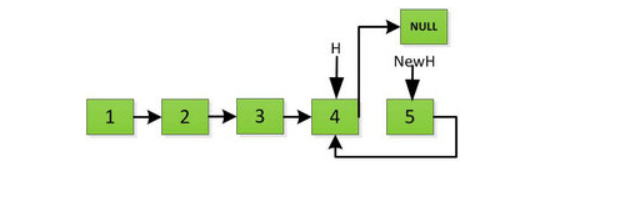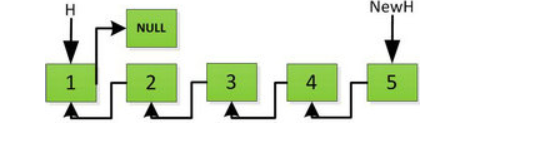<?php
class Node{
public   $next=null; public$data;
public function __construct($data=''){$this->data=$data; } } //新增 function addNode($head,$data){$cur=$head; while($cur->next!=null){
$cur=$cur->next;
}
$node=new Node($data);
$cur->next=$node;

}

//遍历
function showNode($head){$cur=$head; while($cur->next!=null){
$cur=$cur->next;
echo $cur->data.PHP_EOL; } } //feidigui非递归 function reversNode($head){
if($head==null){ return false; }$new=null;
$cur=$head;
while($cur!=null){$temp=$cur->next;$cur->next=$new;$new=$cur;$cur=$temp; } return$new;

}
//递归方式实现
function reversRecursionNode($head){ if($head->next==null){
return $head ; }$phead=reversRecursionNode($head->next);$head->next->next=$head;$head->next=null;
return $phead; } function test(){$head=new Node();
addNode($head,'A'); addNode($head,'B');
addNode($head,'C'); addNode($head,'D');
showNode($head); echo '--------------'; //$new=reversNode($head);$new=reversRecursionNode($head); echo'-----------------'; showNode($new);
echo'------------------------';
$head=new Node();$head->next=$new; showNode($head);
}

test();

?>